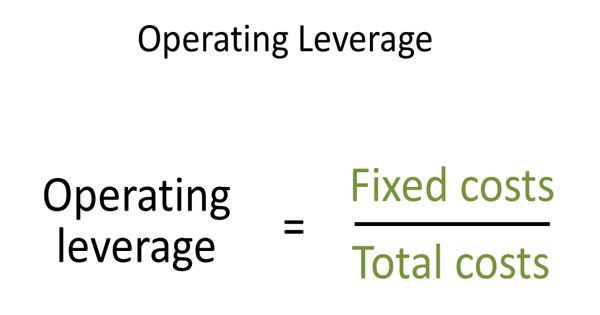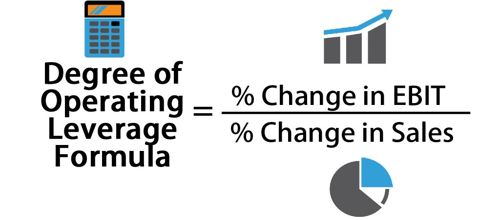Accounting

# Degree of Operating Leverage (DOL)The degree of operating leverage (DOL) is a multiple that measures how much the operating income of a company will change in response to a change in sales. Operating leverage measures the percentage change in operating profit with respect to changes in the sales. The DOL ratio assists analysts in determining the impact of any change in sales on company earnings or profit. The DOL reflects the operating risk a company faces as a result of the structure of its fixed and variable costs. The degree of operating leverage (DOL) is a financial ratio that measures the sensitivity of a company’s operating income to its sales.

The degree of operating leverage (DOL) is a financial ratio that measures the sensitivity of a company’s operating income to its sales.

Therefore, operating leverage is determined through the relationship of the firm’s sales and its operating profit (earnings before interest and tax). A company with high operating leverage has a large proportion of fixed costs, meaning a big increase in sales can lead to outsized changes in profits. The relationship between contribution margin and EBIT is called the degree of operating leverage. It may be defined as the rate of changes in EBIT due to the change in the rate of sales. It measures the sensitivity of the change in operating income (or EBIT, earnings before interest and taxes) to the change in sales revenue. It is used to evaluate a business’ breakeven point—which is where sales are high enough to pay for all costs, and the profit is zero.The degree of operating leverage (DOL) is measured by using any one of the following approaches. The concept of DOL revolves around the proportion of fixed costs and variable costs in the overall cost structure of a company.

Measuring Degree of Operating Leverage (DOL) By Income Statement Approach:

The main formula used to calculate the degree of operating leverage divides the percent change in EBIT by the percent change in sales.

DOL = Contribution Margin/EBIT

Measuring Degree of Operating Leverage By Formula Approach:

DOL = Sales – Variable Cost/Sales – Variable Cost – Fixed Cost

= S-VC/S-VC-FC

Operating Leverage takes into account the proportion of fixed costs to variable costs in the operations of a business. If the degree of operating leverage is high, it means that the earnings before interest and taxes would be unpredictable for the company, even if all the other factors remain the same.

Information Source: